# Schrodinger Wave Equation Assignment Help

1. Home
2. Chemistry
3. Atomic Structure
4. Schrodinger Wave Equation

## 3.14 Schrodinger Wave Equation

The behaviour of an electron inside an atom can be expressed through Schrodinger Wave Equation.y ® wave function

m ® mass of electron

h ® Planck's constant

E ® total energy of electron.

V ® potential energy of electron.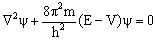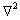is Laplacian Operator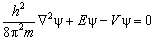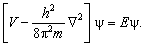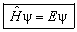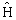is Hamiltonian Operator. It means whenever Hamiltonian operator is applied on the wave function y, we get total energy associated with the wave function, y

(1) Nodes: Probability of finding electron is zero.

Number of radial / spherical nodes = n – l – 1.

More is the number of nodes more is the energy of orbital.

(2) Nodal point: The point at which radial probability becomes zero is known as Nodal Point = n – l

(3) Nodal plane: Number of nodal plane for a subshell = l

## Schrodinger Wave Equation Assignment Help By Online Tutoring and Guided Sessions from AssignmentHelp.Net### Email Based Assignment Help in Schrodinger Wave Equation

Schrodinger Wave Equation is a topic in chemistry atomic structure. If you want Assignment Help homework help in Schrodinger Wave Equation, assignmenthelp.net online tutors is available for you.